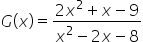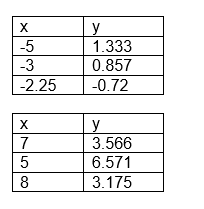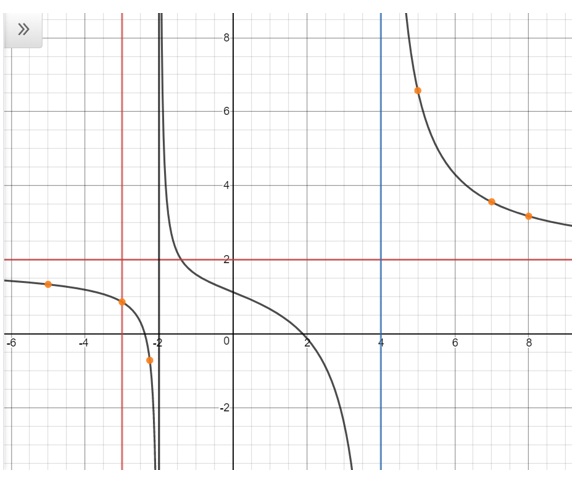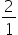Maths-
General
Easy

Question

# What are the vertical and horizontal asymptotes of the graph of each function?Hint:

## The correct answer is: From the graph we can analyze that the vertical asymptote of the rational function is x= -3 and x = -4. and horizontal asymptote is y = (leading coefficient of numerator) / (leading coefficient of denominator) = 2/1 =2

### Find the asymptotes of the rational function, if any. Draw the asymptotes as dotted lines. Find the x -intercept (s) and y -intercept of the rational function, if any. Find the values of y for several different values of x . Plot the points and draw a smooth curve to connect the points. Make sure that the graph does not cross the vertical asymptotes. The vertical asymptote of a rational function is x -value where the denominator of the function is zero. Equate the denominator to zero and find the value of x .x2 - 2x - 8 = 0x2 + 2x - 4x - 8 = 0x(x + 2)- 4(x + 2) = 0(x + 2)(x - 4 )= 0x = -2  or  x = 4The vertical asymptote of the rational function is x =−2 and x = 4This function has x -intercept at (-2.386,0) and y -intercept at (0,1.125) . We will find more points on the function and graph the function.From the graph we can analyze that the vertical asymptote of the rational function is  x= -3 and x = -4. and horizontal asymptote isy = (leading coefficient of numerator) / (leading coefficient of denominator) ==2#### With Turito Foundation.#### Get an Expert Advice From Turito.